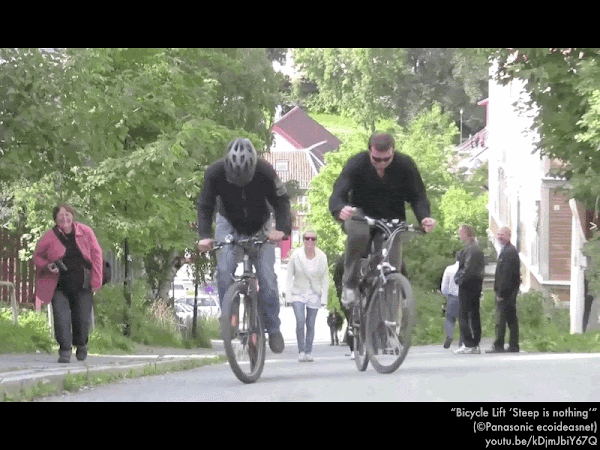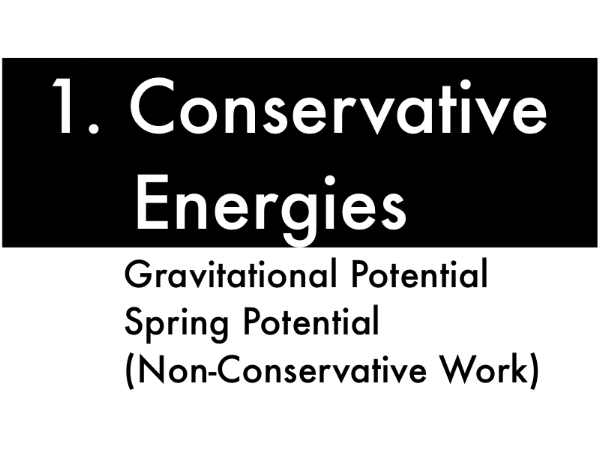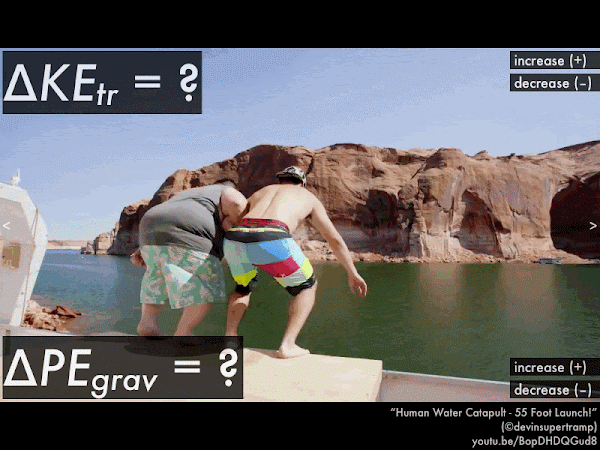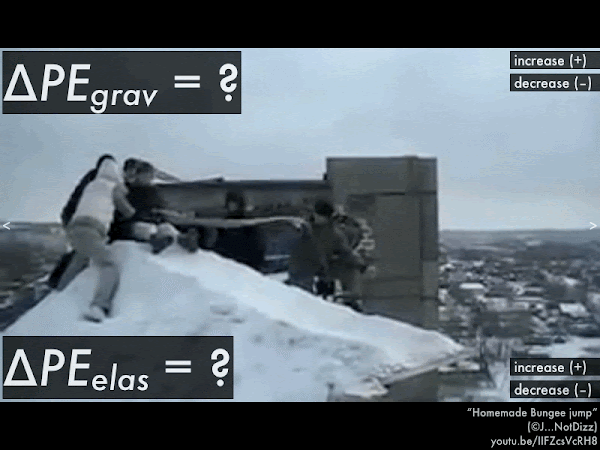## 20170930

### Presentation: energy conservationBike, meet hill. Hill, meet bike lift. Bike lift. (Video link: "Bicycle Lift ‘Steep is nothing’ -Panasonic ecoideasnet.")In this presentation we will complete our introduction to the most common mechanical forms of energy that can be readily transferred amongst each other, along with how this mechanical energy can be irreversibly lost, or irreversibly replenished in the framework of energy conservation.First, a discussion on forces such as the gravitational weight force, and the force of elastic materials and springs, and how the work done by these forces can be stored and later retrieved without loss. Thus these forces are said to be "conservative," as opposed to non-conservative forces that do work that irreversibly loses or gains energy.The weight force of Earth on an object is a conservative force, as when this gravitational force does work against an object, removing its energy, it is able to store this energy, and return it to the object by later doing work on the object. Because of this, instead of explicitly calculating the work done against or an object by the weight force during these storage/retrieval processes, it is much more convenient to talk about increases or decreases in this gravitational "potential" energy.Gravitational potential energy depends on the mass m of the object, the gravitational constant g, and the elevation y of that object. The resulting units of kg·m2/s2 are equivalent to joules. An object on the ground has zero gravitational potential energy, and the higher elevation it has, the more gravitational potential energy it has.

Instead of finding out how much gravitational potential energy PEgrav an object has, often we are more concerned with its initial-to-final change ∆PEgrav, which is the final amount of gravitational potential energy minus the initial amount of gravitational potential energy. Notice how the common factors of m and g (which are presumed to be constant) are pulled out, leaving a "difference of elevations" for the final and initial values of y in the parenthesis.The elastic force of "stretchy" material such as rubber tubing (as in this slingshot), or springs is another conservative force, as when this elastic force does work against an object, removing its energy, it is able to store this energy, and return it to the object by later doing work on the object. Because of this, instead of explicitly calculating the work done against or an object by an elastic force during these storage/retrieval processes, it is much more convenient to talk about increases or decreases in this elastic "potential" energy.Elastic potential energy depends on the elastic/spring constant k (which is a measure of how difficult it is to stretch/compress) and the distance x that the elastic/spring is stretched/compressed from its relaxed equilibrium (where x is set to be zero). The resulting units of N·m are equivalent to joules. A relaxed elastic/spring contains zero elastic potential energy, and the further it is stretched or compressed from its relaxed state, the more elastic potential energy it has.

Instead of finding out how much elastic potential energy PEelas an object has, often we are more concerned with its initial-to-final change ∆PEelas, which is the final amount of elastic potential energy minus the initial amount of elastic potential energy. Notice how the common factors of (1/2) and elastic/spring constant k (which is presumed to be constant) are pulled out, leaving a "difference of squares" for the final and initial values of x in the parenthesis.Keep in mind that gravitational potential energy and elastic potential energy involve the conservative forces of weight and of elastic materials, which ideally work against or on objects to receive, store, and return energy without loss. Combined with translational kinetic energy KEtr, PEgrav and PEelas are the useful "mechanical" forms of energy that can be readily transferred between each form for current or later use.

In contrast, when non-conservative forces are exerted against the motion of objects, energy is lost, irreversibly converted to non-mechanical forms; or when non-conservative forces work along the motion of objects, energy is gained, but this is from the irreversible conversion of non-mechanical forms.

Here we have many examples of non-conservative forces acting on the cat. As it slides across the floor, friction and drag act against the direction of motion of the cat, removing its translational kinetic energy, and it eventually slows to a stop. This energy is irreversibly lost, dissipated to heating up the cat, contact surfaces, and stirring up the air in the room, and cannot be recovered.

Also the people at either end of the kitchen push on the cat along its direction of motion, adding to its translational kinetic energy. This energy is irreversibly converted from the biochemical reactions in the people, and cannot be recovered.

Because these non-conservative forces involve irreversible transfers to/from mechanical energy forms, we must explicitly account for the non-conservative work done by them in energy conservation accounting.Second, accounting for the transfers between different mechanical energy forms, and also for the contribution to or taking from these energies by non-conservative work.In contrast to the "incomplete" work-energy theorem, this is the complete form of total energy conservation equation, which shows how the transfer of energy (as work is done by non-conservative forces acting on or against the motion of the object) causes a corresponding change in the translational kinetic energy of the object, along with the potential gravitational energy and the potential elastic energy. Any or all of these energy forms on the right-hand side of the equation can increase or decrease, but together all of their changes must add up to the corresponding non-conservative work term on the left-hand side of this equation.

Note that in the idealized case that there are no non-conservative forces (such as friction, drag, or from external sources), then the left-hand size of this equation would be zero. Then the individual energy terms on the right-hand side of this equation can then trade and balance amongst themselves, instead of with the outside world.

So now let's see how this transfer/balance equation can be applied to idealized situations where friction and drag are negligible.Let's look at the transfer/balance equation terms for a woman launched by this "human water catapult." Her initial state is just after being launched (and moving upwards with a fast speed); and her final state is at her highest height (where she is momentarily stationary, before coming back down). (Video link: "Human Water Catapult - 55 Foot Launch! In 4k (HD).")

From just after being launched, to reaching her highest height, as the woman is moving upwards her speed is decreasing, and since translational kinetic energy depends on the square of her speed, as her speed decreases, her translational kinetic energy decreases. (If you calculated her change in translational kinetic energy you would get a negative value, which is consistent with a decrease.)

From just after being launched, to just before reaching her highest height, as the woman is moving upwards her height is increasing, and since gravitational potential energy depends on height, as her height increases, her gravitational potential energy increases. (If you calculated her change in gravitational potential energy you would get a positive value, which is consistent with an increase.)

Since we are assuming friction and drag are negligible, then there is no non-conservative work done on the woman, so the left-hand side of the transfer/balance equation is zero:

Wtr = ∆KEtr + ∆PEgrav + ∆PEelas,

0 = ∆KEtr + ∆PEgrav + ∆PEelas,

and since there are no changes in elastic potential energy while the woman is already moving upwards in the air, we are only left with the changes in the translational kinetic energy and gravitational potential energy terms:

0 = ∆KEtr + ∆PEgrav + 0,

0 = ∆KEtr + ∆PEgrav,

0 = (+) + (–).

Now we can see that amount that the woman's translational kinetic energy decreases (where ∆KEtr is negative) is directly related to the amount that the woman's gravitational potential energy increases (where ∆PEgrav is positive), in order to equal the zero on the left-hand side of the equation. So translational kinetic energy "feeds" (or is "transferred to") gravitational potential energy during this process.Now for this ball bearing being launched by this slingshot, let's have its initial state as when it is at rest and the elastic bands are fully stretched; and the final state is when the elastic bands are slack with the ball bearing at full speed. (Note that if the slingshot is aimed horizontally, then there is no change in height for the ball bearing, so its gravitational potential energy does not change.) During this process, does the ball bearing's translational kinetic energy increase or decrease? Does the elastic potential energy of the slingshot bands increase or decrease?

Assuming that there is no friction/drag (such that we can neglect non-conservative work), is energy being transferred from translational kinetic energy to elastic potential energy, or is it transferred the other way around? (Video link: "The Physics of Slingshots 2 | Smarter Every Day 57.")Then for this woman bungee jumper, let's have her initial state as when she is at rest (just before beginning to slide) on top of the roof, with a slack bungee cord; and her final state is when the bungee cord is fully stretched as she swings through her lowest point of descent. For this process, does her translational kinetic energy increase or decrease? Does her gravitational potential energy increase or decrease? Does the elastic potential energy of the bungee cord increase or decrease?

Assuming that there is no friction/drag (such that we can neglect non-conservative work), which energy system is "feeding" the others? Which energy system experienced a greater amount of change (increase or decrease): translational kinetic energy, gravitational potential energy or the elastic potential energy? (Video link: "Homemade Bungee jump.")